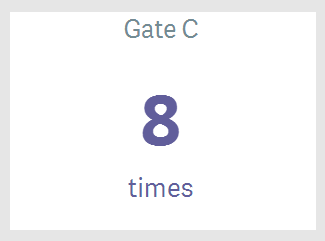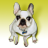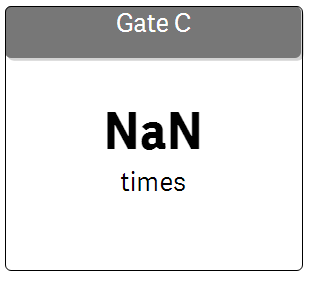# New to Qlik Sense

If you’re new to Qlik Sense, start with this Discussion Board and get up-to-speed quickly.

Announcements
QlikWorld 2023, a live, in-person thrill ride. Save \$300 before February 6: REGISTER NOW!
cancel
Showing results for
Did you mean:Creator

## How can I display result which is greater than 0?

Dear Friend,

My result display value which include 0 & negative. how to modified my formula to exclude it? Thanks.

Have a nice day 🙂

=Count({\$<[SR No]={"=Min ({<Temp = {'Task_1'}>}[Date Created]) >=  MakeDate(2018,1,15)"}>

*\$<[SR No]-={"''"}>

*\$<[Gate]={"Gate C"}>

}

(Gate))-count([SR No])

1 Solution

Accepted SolutionsCreator
Author

Dear All,

sum(Aggr(

if(

(Count({\$<[SR No]={"=Min ({<Temp = {'Task_1'}>}[Date Created]) >=  MakeDate(2018,1,15)"}>

*\$<[SR No]-={"''"}>

*\$<[Gate]={"Gate C"}>

}(Gate))-count([SR No]))>0,

(Count({\$<[SR No]={"=Min ({<Temp = {'Task_1'}>}[Date Created]) >=  MakeDate(2018,1,15)"}>

*\$<[SR No]-={"''"}>

*\$<[Gate]={"Gate C"}>

}(Gate))-count([SR No])),

'No repetition') , [SR No]))6 RepliesPartner - Creator II

Put the expression in a variable and use an if condition?Creator
Author

Hi Arjun,

I manage to get the display in table. but how im gonna show the total in KPI object? it showing NaN.Could be due to the text. it should  show 8 instead of NaN.

if(

(Count({\$<[SR No]={"=Min ({<Temp = {'Task_1'}>}[Date Created]) >=  MakeDate(2018,1,15)"}>

*\$<[SR No]-={"''"}>

*\$<[Gate]={"Gate C"}>

}(Gate))-count([SR No]))>0,

(Count({\$<[SR No]={"=Min ({<Temp = {'Task_1'}>}[Date Created]) >=  MakeDate(2018,1,15)"}>

*\$<[SR No]-={"''"}>

*\$<[Gate]={"Gate C"}>

}(Gate))-count([SR No])),

'No repetition')Partner - Master III

i think you'll need an aggr function to achieve it.MVP

from;

YourMeasure

to:

if (YourMeasure>0, YourMeasure, 0)

and under add-on, uncheck : show zro values.MVP

in a KPI, try sthing like:

sum({<Yourdim={"=sum(YourMeasure)>0"}>}YourMeasure)Creator
Author

Dear All,

sum(Aggr(

if(

(Count({\$<[SR No]={"=Min ({<Temp = {'Task_1'}>}[Date Created]) >=  MakeDate(2018,1,15)"}>

*\$<[SR No]-={"''"}>

*\$<[Gate]={"Gate C"}>

}(Gate))-count([SR No]))>0,

(Count({\$<[SR No]={"=Min ({<Temp = {'Task_1'}>}[Date Created]) >=  MakeDate(2018,1,15)"}>

*\$<[SR No]-={"''"}>

*\$<[Gate]={"Gate C"}>

}(Gate))-count([SR No])),

'No repetition') , [SR No]))Tags
Community Browser